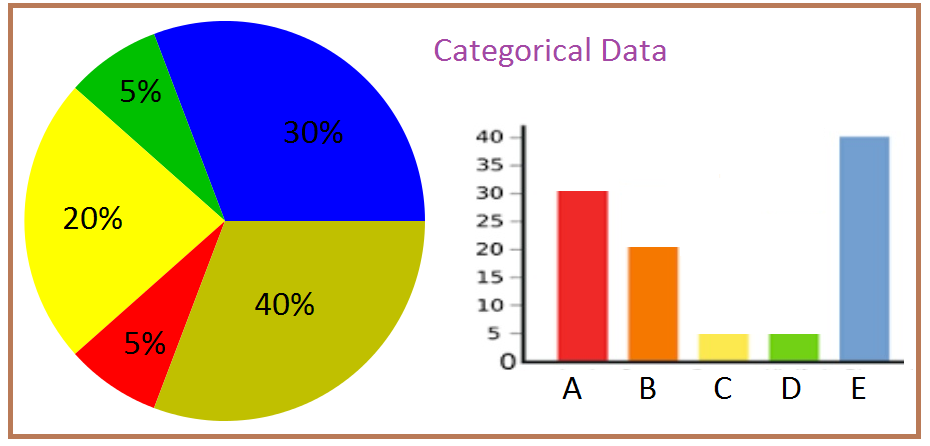Checkout JEE MAINS 2022 Question Paper Analysis : Checkout JEE MAINS 2022 Question Paper Analysis :

# Categorical Data

Categorical data is the statistical data comprising categorical variables of data that are converted into categories. One of the examples is a grouped data. More precisely, categorical data could be derived from qualitative data analysis that are countable, or from quantitative data analysis grouped within given intervals. These data are summarised in the form of a probability table. However, when we consider data analysis, it is referred to use the term “categorical data”, which is applied to data sets. Also, it is to be noted that, while containing some categorical variables, the data set may also contain non-categorical variables.

In statistics, it is important to recognise the different types of data. It is because statistical methods can be done only with the help of data types. Having knowledge of different kinds of data helps you to analyse the correct method. Data are the actual pieces of information that are collected through the study. It is observed that most of the data fall under two groups namely,

• Numerical Data or Quantitative data
• Categorical Data or Qualitative Data

Now, let us take detailed information on categorical data in statistics.

## Categorical or Qualitative Data

The categorical data consists of categorical variables which represent the characteristics such as a person’s gender, hometown etc. Categorical measurements are expressed in terms of natural language descriptions, but not in terms of numbers. Sometimes categorical data can take numerical values, but those numbers do not have mathematical meaning. Some of the examples of the categorical data are as follows:

• Birthdate
• Favourite sport
• School Postcode
• Travel method to school etc.

When you observe the above example, birthdate and postcode contain numbers. Even though it contains numerals, it is considered as categorical data. The easy way to determine whether the given data is categorical or numerical data is to calculate the average. If you are able to calculate the average, then it is considered to be a numerical data. If you cannot calculate the average, then it is considered to be a categorical data. Like the example mentioned above, the average of birthdate and the postal code has no meaning, so it is taken as categorical data.

## Types of Categorical Data

In general, categorical data has values and observations which can be sorted into categories or groups. The best way to represent these data is bar graphs and pie charts. Categorical data are further classified into two types namely,

• Nominal Data
• Ordinal Data

### Nominal Data

Nominal data is a type of data that is used to label the variables without providing any numerical value. It is also known as the nominal scale. Nominal data cannot be ordered and measured. But sometimes nominal data can be qualitative and quantitative. Some of the few common examples of nominal data are letters, words, symbols, gender etc.

These data are analysed with the help of the grouping method. The variables are grouped together into categories and the percentage or frequency can be calculated. It can be presented visually using the pie chart.

### Ordinal Data

Ordinal data is a type of data that follows a natural order. The notable features of ordinal data are that the difference between data values cannot be determined. It is commonly encountered in surveys, questionnaires, finance and economics.

The data can be analysed using visualisation tools. It is commonly represented using a bar chart. Sometimes the data may be represented using tables in which each row in the table indicates the distinct category.## Categorical Variables

In statistics, a categorical variable is a variable that contains limited, and usually a fixed number of possible values. They take values which are normally names or labels. Examples are:

• The colour of a wall, like red, blue, pink, gree, etc.,
• Gender of people, like male, female and transgender
• Blood group of a person: A, B, O, AB, etc.,

These variables are used to assign each individual or another unit of observation to a particular group or nominal category based on some qualitative property. Generally, each of the potential values of a categorical variable is said to be as a level. The probability distribution linked with a random categorical variable is known as categorical distribution.

## Categorical and Numerical Data

• Categorical data or Qualitative data consist of categorical values or variables, where the data are represented in labelled or given a name. Such as the breed of a dog, colour of the car, and so on
• Numerical data or Quantitative data comprising numbers or numerical values to represent the data, such as height, weight, age of a person

Register with BYJU’S – The Learning App for more such information on statistical data types and also watch other maths-related articles.